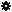﻿ Object Model: VertexCollection.NeighborEdges

VertexCollection.NeighborEdges

## VertexCollection.NeighborEdgesv3.0

### Description

Returns a collection of Edge objects for all neighbors within the specified distance.

### C# Syntax

 ```EdgeCollection VertexCollection.NeighborEdges( Int32 in_lDistance ); ```

### Scripting Syntax

 ```oReturn = VertexCollection.NeighborEdges( [Distance] ); ```

### Parameters

Parameter Type Description
Distance Integer Distance is an integer value representing the degree of neighborhood (eg: degree=2 for a vertex means its adjacent vertices plus the adjacent vertices of the adjacent vertices)

Default Value: 1

### Examples

#### VBScript Example

 ```set oCube = ActiveSceneRoot.AddGeometry( "Cube", "MeshSurface" ) set oGeometry = oCube.ActivePrimitive.Geometry set oPolygons = oGeometry.Polygons set oNeighborVertices = oPolygons(0).NeighborVertices(1) set oNeighbors = oNeighborVertices.NeighborEdges str = "Edges neighbors of this EdgeCollection are :" for each n in oNeighbors str = str & " " & n.index next logmessage str ```